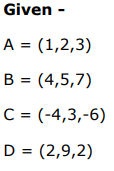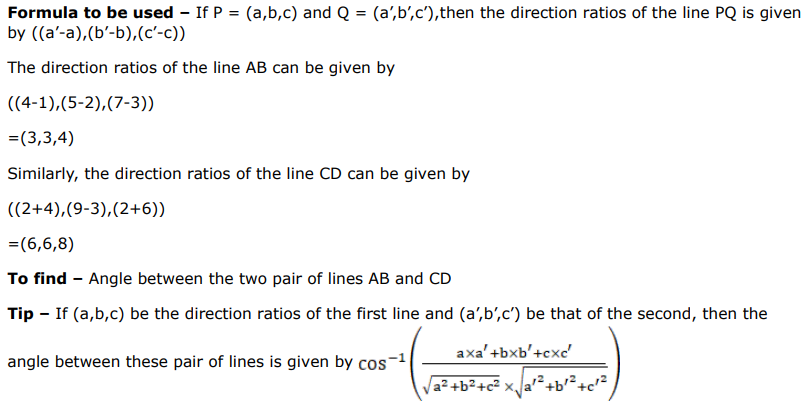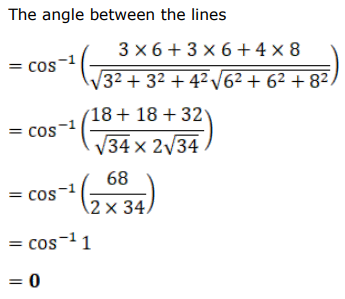# Solve this following

Question:

If $A(1,2,3), B(4,5,7), C(-4,3,-6)$ and $D(2,9,2)$ are four given points then find the angle between the lines $A B$ and $C D$.

Solution: